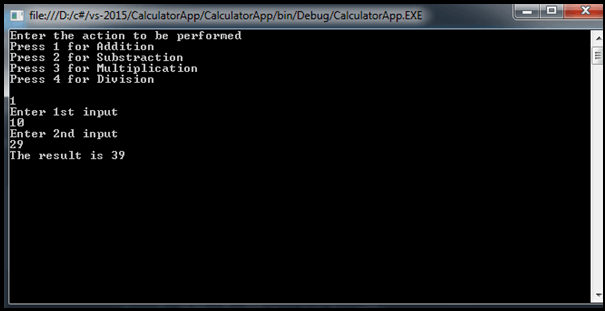# Write a C# program to create a Simple Calculator

Write a program to create a Simple Calculator in C#. In this article, we will learn to create a simple calculator in C# using ‘select case’. Here, we will perform all the arithmetic operations.

The given program is compiled and executed successfully. Here is the complete code of the app written in C#. In this program, there are four functional blocks–Addition, Subtraction, Multiplication, and Division. The user has to select one of the options.

## C# Code for Simple Calculator

```using System;
using System.Collections.Generic;
using System.Linq;
using System.Text;
namespace CalculatorApp {
class Program {
static void Main(string[] args) {
Console.WriteLine("Enter the action to be performed");
Console.WriteLine("Press 2 for Subtraction");
Console.WriteLine("Press 3 for Multiplication");
Console.WriteLine("Press 4 for Division \n");
Console.WriteLine("Enter 1st input");
Console.WriteLine("Enter 2nd input");
int result = 0;
switch (action) {
case 1: {
break;
}
case 2: {
result = Subtraction(input_1, input_2);
break;
}
case 3: {
result = Multiplication(input_1, input_2);
break;
}
case 4: {
result = Division(input_1, input_2);
break;
}
default:
Console.WriteLine("Wrong action!! try again");
break;
}
Console.WriteLine("The result is {0}", result);
}
public static int Addition(int input_1, int input_2) {
int result = input_1 + input_2;
return result;
}
//Substraction
public static int Subtraction(int input_1, int input_2) {
int result = input_1 + input_2;
return result;
}
//Multiplication
public static int Multiplication(int input_1, int input_2) {
int result = input_1 + input_2;
return result;
}
//Division
public static int Division(int input_1, int input_2) {
int result = input_1 + input_2;
return result;
}
```

Explanation:

In the above program, we have a Main() method. Inside it, we have four variables action, input_1, input_2, and result.

In the above program, we defined “select case” to perform the operation. Here we select 1st case and perform the addition operation and print the result on the console screen.

Working:

In the above program firstly the compiler check for the main method then it prints the choices which are given in the console.Writeline() as follows:

```press 1 for addition,
press 2 for subtraction,
press 3 for multiplication,
press 4 for division.```

After that, we have to choose 1 option from these which is a select case variable whose name is ‘action’ we take input in this variable by “int action = Convert.ToInt32(Console.ReadLine());”

The readline() is used to take user input from the keyboard and the number we have selected if any given select case is matched with it so that select case statement body will run otherwise the default case will run which shows “Wrong action!! try again”.

Also, there are 4 modules that take the input as arguments whichever module will call in switch case that module body will run and it will put the inputs in its argument parameter and do the calculations on it.

A Select Case block evaluates action to determine which operator has been invoked and then applies the operator to input_1 and input_2 to calculate the value of the result.

### Output screenIn this way, we create a Simple Calculator in C#. In case you want to know How to create a Simple Calculator Program in VB.NET check this article https://codebun.com/write-a-program-to-create-a-simple-calculator-in-visual-basic-vb-net/